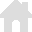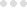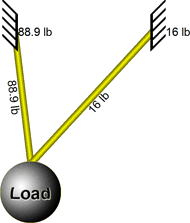vRigger Learning Center

This also applies to cable, chain, and webbing.

Gear that is anchored includes anchors, rocks, trees, tripods, trucks, etc.

A "bight" is a simple loop in a rope that does not cross itself.

A "bend" is a knot that joins two ropes together. Bends can only be attached to the end of a rope.

"Descending devices" (e.g., ATCs, Brake Bar Racks, Figure 8s, Rescue 8s, etc) create friction as their primary purpose. The friction in descending devices is always considered when calculating forces.

The "Safety Factor" is the ratio between the gear's breaking strength and the maximum load applied to the gear (e.g., 5:1).

# Statically Indeterminate ForcesIn some rigging systems, an infinite number of solutions are possible when determining how forces are shared between two or more pieces of gear. These systems are referred to as "statically indeterminate."

For example, it is clear in the system to the right that the load's 100 pounds must be shared between the two anchors, but it isn't clear how much of the 100 pounds should be assigned to each of the anchors.

In this solution, vRigger assigned 88.9 pounds to one anchor and 16 pounds to the other. This is a valid solution (when you consider the rope angles), but there are an infinite number of other solutions.

This situation, where it is not clear how forces will be distributed between two or more parts of a system, occurs in the real world regularly. vRigger will stop calculating forces (and stop moving the load horizontally) when it finds the first valid solution. As in the "real" world, if you want the anchors to share the same load, you must equalize the system.